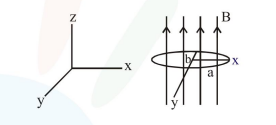# An elliptical loop having resistance

Question:

An elliptical loop having resistance $\mathrm{R}$, of semi major axis a, and semi minor axis b is placed in a magnetic field as shown in the figure. If the loop is rotated about the $x$-axis with angular frequency $\omega$, the average power loss in the loop due to Joule heating is :1. $\frac{\pi^{2} \mathrm{a}^{2} \mathrm{~b}^{2} \mathrm{~B}^{2} \omega^{2}}{2 \mathrm{R}}$

2. Zero

3. $\frac{\pi^{2} \mathrm{a}^{2} \mathrm{~b}^{2} \mathrm{~B}^{2} \omega^{2}}{\mathrm{R}}$

4. $\frac{\pi \mathrm{abB} \omega}{\mathrm{R}}$

Correct Option: 1

Solution:

$\epsilon=\mathrm{NAB} \omega \cos \omega t \quad \mathrm{~N}=1$

$\mathrm{P}_{\text {avg }}=\left\langle\frac{\epsilon^{2}}{\mathrm{R}}\right\rangle=\left\langle\frac{(\mathrm{AB} \omega \cos \omega \mathrm{t})^{2}}{\mathrm{R}}\right\rangle$

$=\frac{\mathrm{A}^{2} \mathrm{~B}^{2} \omega^{2}}{\mathrm{R}} \frac{1}{2}=\frac{\pi^{2} \mathrm{a}^{2} \mathrm{~b}^{2} \mathrm{~B}^{2} \omega^{2}}{2 \mathrm{R}}$